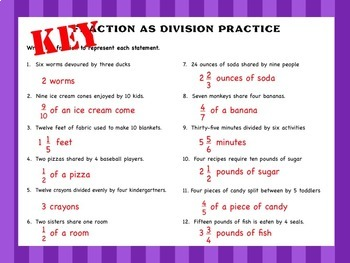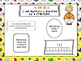# Multiplying Fractions Mega Bundle – Distance LearningSubject
Resource Type
File Type

Zip

(149 MB|58 pages)
Standards
3 Products in this Bundle
3 products
1. Multiplying a Fraction by a Fraction Pack – This complete multiplying a fraction by a fraction practice pack includes everything you will need to create a fun and engaging learning opportunity for students. Included in this Packet • Pre and Post Assessments with Keys • Objective Poster • Interac
2. Multiplying a Fraction by a Whole Number Pack – This complete multiplying a fraction by a whole number practice pack includes everything you will need to create a fun and engaging learning opportunity for students. Included in this Packet • Pre and Post Assessments with Keys • Objective Poster •
3. Multiplication as Scaling Practice Pack – This complete multiplication as scaling practice pack includes everything you will need to create a fun and engaging learning opportunity for students. Included in this Packet • Pre and Post Assessments with Keys • Objective Poster • Interactive Notes a
• Bundle Description
• StandardsNEW
Multiplying Fractions Bundle Pack – This bundle includes everything you will need to teach multiplying fractions to your fourth or fifth grade class.

This unit includes
• Fractions as Division Pack
• Multiplying a Fraction by a Whole Number Pack
• Multiplying a Fraction by a Fraction Pack

Each Pack Includes
• Pre and Post Assessments with Keys
• An Objective Poster
• Interactive Notes and Foldables
• Student Samples and Instructions
• 3 Different Practice Pages and Games with Answer Keys

********Great Addition to Interactive Notebooks or Centers********

Interactive Notebooks
Interactive notebooks are a great way for students to refer back to notes they have taken in class. Students can easily integrate the resources from this pack into their notebooks.

Centers
The foldables and practice pages in this pack can be incorporated into centers where students rotate from one station to another. At this station, students can either work independently or in pairs.

Standards:

5.NF.B.3 Interpret a fraction as division of the numerator by the denominator (a/b = a ÷ b). Solve word problems involving division of whole numbers leading to answers in the form of fractions or mixed numbers, e.g., by using visual fraction models or equations to represent the problem.

5.NF.B.4 Apply and extend previous understandings of multiplication to multiply a fraction or whole number by a fraction.

4.NF.B.4 Apply and extend previous understandings of multiplication to multiply a fraction by a whole number.

* * * * * * * * * * * * * * * * * * * * * * * * * * * * * * * *
CHECK OUT THESE OTHER MULTIPLYING FRACTIONS PACKS (click on each to view):

Fractions as Division PackMultiplying a Fraction by a Fraction PackMultiplying a Fraction by a Whole Number PackMultiplication as Scaling PackFraction Wheel Game

* * * * * * * * * * * * * * * * * * * * * * * * * * * * * * * *

Please click the "Follow Me" link located under my picture and store name on the right-hand column of this page to keep updated on all my new offerings!

Find the area of a rectangle with fractional side lengths by tiling it with unit squares of the appropriate unit fraction side lengths, and show that the area is the same as would be found by multiplying the side lengths. Multiply fractional side lengths to find areas of rectangles, and represent fraction products as rectangular areas.
Interpret the product (𝘢/𝘣) × 𝘲 as a parts of a partition of 𝘲 into 𝘣 equal parts; equivalently, as the result of a sequence of operations 𝘢 × 𝘲 ÷ 𝘣. For example, use a visual fraction model to show (2/3) × 4 = 8/3, and create a story context for this equation. Do the same with (2/3) × (4/5) = 8/15. (In general, (𝘢/𝘣) × (𝘤/𝘥) = 𝘢𝘤/𝘣𝘥.)
Apply and extend previous understandings of multiplication to multiply a fraction or whole number by a fraction.
Interpret a fraction as division of the numerator by the denominator (𝘢/𝘣 = 𝘢 ÷ 𝘣). Solve word problems involving division of whole numbers leading to answers in the form of fractions or mixed numbers, e.g., by using visual fraction models or equations to represent the problem. For example, interpret 3/4 as the result of dividing 3 by 4, noting that 3/4 multiplied by 4 equals 3, and that when 3 wholes are shared equally among 4 people each person has a share of size 3/4. If 9 people want to share a 50-pound sack of rice equally by weight, how many pounds of rice should each person get? Between what two whole numbers does your answer lie?
Solve word problems involving addition and subtraction of fractions referring to the same whole and having like denominators, e.g., by using visual fraction models and equations to represent the problem.
Total Pages
58 pages
Included
Teaching Duration
2 Weeks
Report this Resource to TpT
Reported resources will be reviewed by our team. Report this resource to let us know if this resource violates TpT’s content guidelines.